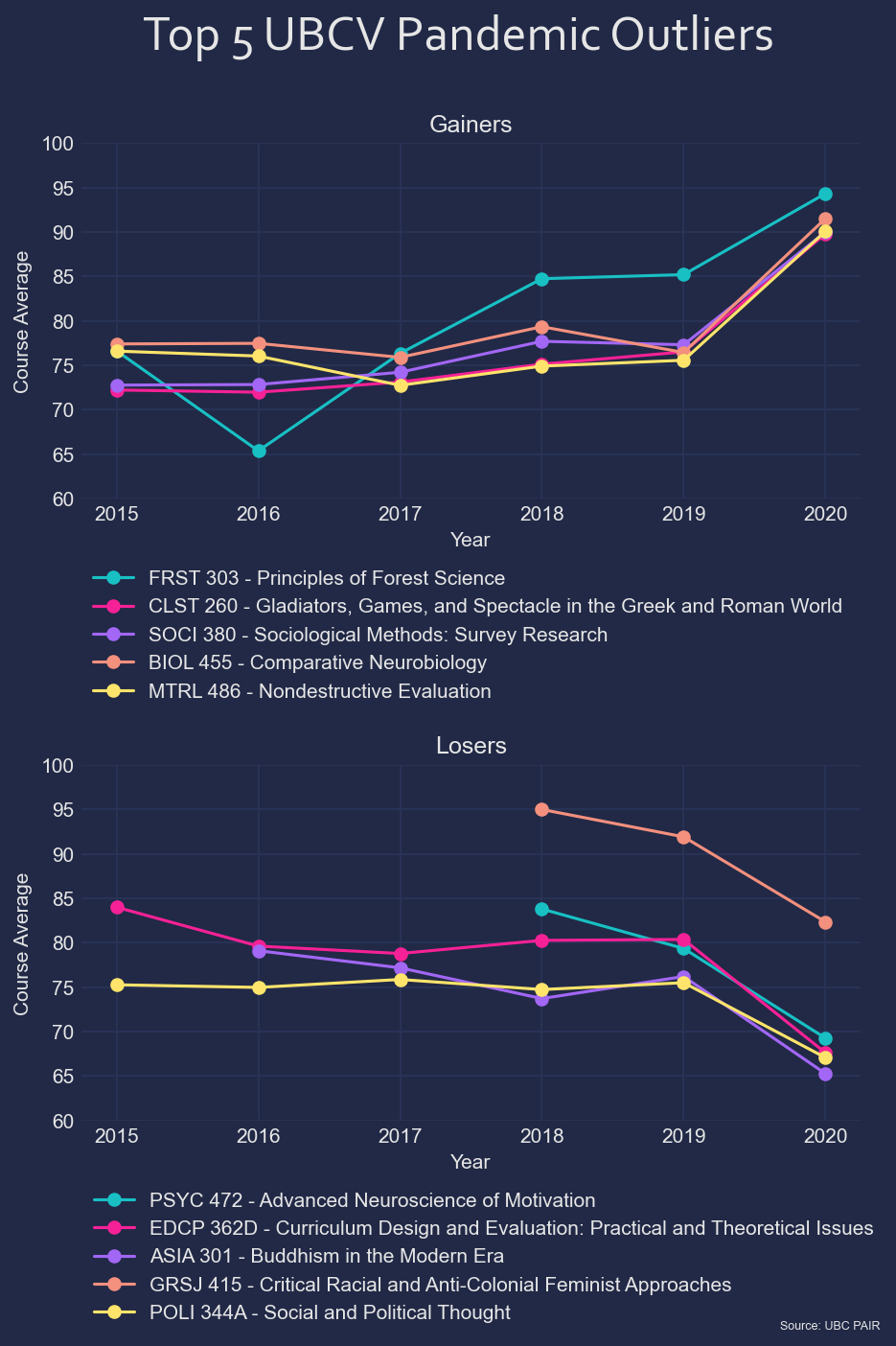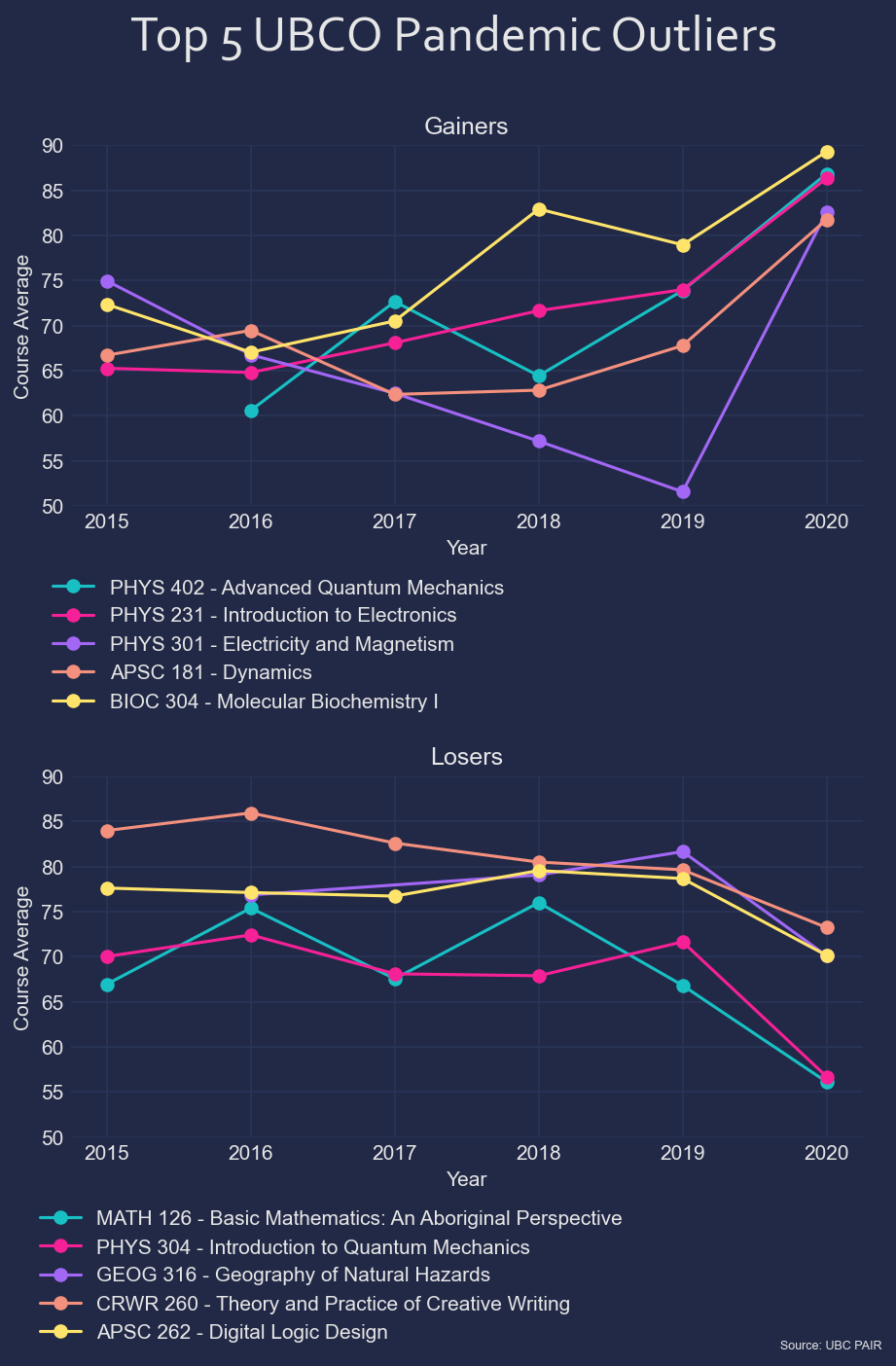Imports¶

In :
import numpy as np
import pandas as pd
import csv
import json
import os

Import CSV to pd¶

You'll need to git clone the repo here.

In :
In :
dataframes = []

for dirpath, subdirs, csv_files in os.walk(path_to_csv_files):
for csv_file in csv_files:
In :
df = pd.concat(dataframes, ignore_index=True)

Analyze¶

In :
# Drop summer sessions
df = df[~df['Session'].str.contains('S')]
In :
# Keep only OVERALL sections
df = df[df['Section'].str.contains('OVERALL')]
In :
# Create unique keys course and detail into one column
df['Key'] = df['Campus'] + '-' + df['Subject'] + '-' + df['Course'].astype(str) + df['Detail'].fillna('').astype(str)
In :
# Get other years
df2020 = df[(df['Year'] == 2020)]
df2019 = df[(df['Year'] == 2019)]
df2018 = df[(df['Year'] == 2018)]
In [ ]:
# Remove rows from 2020 if the change from 2018 to 2019 was not significant. Begin by computing the difference
df2019['diff_2019_2018'] = df2019['Avg'] - df2019['Key'].map(df2018.set_index('Key')['Avg'])
# Remove NAN values (removes courses that were not offered in 2018)
df2019 = df2019[df2019['diff_2019_2018'].notna()]
# Remove values if abs val is large
df2019 = df2019[np.abs(df2019['diff_2019_2018']) < 10]

# Give those differences to the 2020 dataframe
df2020['diff_2019_2018'] = df2020['Key'].map(df2019.set_index('Key')['diff_2019_2018'])
# Remove NAN values (removes rows from df2020 that were filtered out eaerlier)
df2020 = df2020[df2020['diff_2019_2018'].notna()]
# Remove values if the class size is small
df2020 = df2020[df2020['Enrolled'] >= 15]
In :
# We also want to avoid including courses that have a wide spread of averages. If the 2020 average is not more than 5% away from any previous
# average, do not include it
df_no_2020 = df[~(df['Year'] == 2020)]
threshold = 5

def is_an_outlier(row, df_no_2020):
df_key = df_no_2020[df_no_2020['Key'] == row['Key']]
curr_avg = row['Avg']
max_avg = df_key['Avg'].max()
min_avg = df_key['Avg'].min()
if curr_avg > max_avg:
# Gainer
return curr_avg - threshold > max_avg
elif curr_avg < min_avg:
# Loser
return curr_avg + threshold < min_avg
else:
# Uninteresting
return False

idx = df2020.apply(is_an_outlier, df_no_2020=df_no_2020, axis=1)
df2020 = df2020[idx]
In :
# Define a lambda function to compute the weighted mean:
wm = lambda x: np.average(x, weights=df.loc[x.index, "Enrolled"])

df_weighted = df_no_2020.groupby(['Key']).agg(Avg=("Avg", wm))
In :
df_weighted = df_weighted.reset_index()
In :
df_2020 = df2020.reset_index()
In :
# Subtract the weighted avg from 2020 avg only where the course was offered in 2020
df_diff = df2020
df_diff['Difference'] = df2020['Avg'] - df2020['Key'].map(df_weighted.set_index('Key')['Avg'])
In :
# Any NaN entries in 'Difference' indicates that the course is new for 2020. Drop it.
df_diff = df_diff[~df_diff['Difference'].isnull()]
In :
a = df_diff[df_diff['Campus'] == 'UBCV']
a.loc[a['Difference'].idxmax()]
Out:
Campus                                    UBCV
Year                                      2020
Session                                      W
Subject                                   FRST
Course                                     303
Detail                                     NaN
Section                                OVERALL
Title             Principles of Forest Science
Professor                                  NaN
Enrolled                                   170
Avg                                  94.341176
Std dev                                6.84322
High                                     100.0
Low                                       50.0
<50                                        0.0
50-54                                      1.0
55-59                                      0.0
60-63                                      0.0
64-67                                      1.0
68-71                                      0.0
72-75                                      3.0
76-79                                      1.0
80-84                                      6.0
85-89                                     15.0
90-100                                   143.0
Key                              UBCV-FRST-303
diff_2019_2018                        0.459677
Difference                           18.154233
Name: 97004, dtype: object

Plots¶

In :
import matplotlib.pyplot as plt
from matplotlib.ticker import MaxNLocator
In :
def create_plot(df, gainers, losers, campus, ylims, legend_offsets):
plt.style.use('https://github.com/dhaitz/matplotlib-stylesheets/raw/master/pitayasmoothie-dark.mplstyle')
f, axes = plt.subplots(2,1, figsize=(7, 9), dpi=150)
csfont = {'fontname':'Corbel'}
f.suptitle(f"Top 5 {campus} Pandemic Outliers", fontsize=25, **csfont)

ax = axes
ax.set_ylim(ylims)
ax.title.set_text('Gainers')
ax.set_ylabel('Course Average')
chart_data = df[(df['Key'] == row['Key']) & (df['Year'] >= 2015)]
splits = row['Key'].split('-')
label = ' '.join([splits, splits]) + ' - ' + row['Title']
chart_data.plot(x='Year', y='Avg', ax=ax, marker='o', label=label)

ax.legend(bbox_to_anchor=legend_offsets, bbox_transform=ax.transAxes)

ax = axes
ax.set_ylim(ylims)
ax.title.set_text('Losers')
ax.set_ylabel('Course Average')
ax = axes
chart_data = df[(df['Key'] == row['Key']) & (df['Year'] >= 2015)]
splits = row['Key'].split('-')
label = ' '.join([splits, splits]) + ' - ' + row['Title']
chart_data.plot(x='Year', y='Avg', ax=ax, marker='o', label=label)

ax.legend(bbox_to_anchor=legend_offsets, bbox_transform=ax.transAxes)

plt.annotate('Source: UBC PAIR', (0,0), (350,-100), fontsize=6,
xycoords='axes fraction', textcoords='offset points', va='top')

ax.xaxis.set_major_locator(MaxNLocator(integer=True))
plt.savefig(f'{campus}.jpg',  dpi=f.dpi, bbox_inches = 'tight', pad_inches=0.2)
plt.show()
In :
UBCV = df_diff[df_diff['Campus'] == 'UBCV']
gainers = UBCV.sort_values('Difference', ascending=False)
losers = UBCV.sort_values('Difference')
create_plot(df, gainers, losers, 'UBCV', [60, 100], [(1, -0.15), (1.04,-0.15)]);In :
UBCO = df_diff[df_diff['Campus'] == 'UBCO']
gainers = UBCO.sort_values('Difference', ascending=False)
losers = UBCO.sort_values('Difference')
create_plot(df, gainers, losers, 'UBCO', [50, 90], [(0.57, -0.15), (0.72,-0.15)])